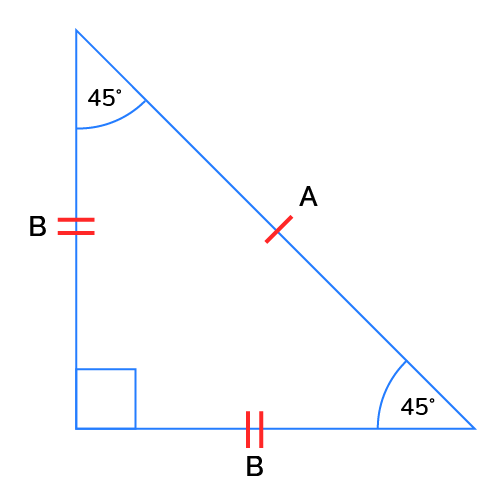# Isosceles Right Triangle Hypotenuse Calculator

Reviewed by Steven Wooding
Last updated: Jun 03, 2022

Are you on the market for an isosceles right triangle hypotenuse calculator? Then look no further. Our isosceles right triangle hypotenuse calculator will help you to find the length of the hypotenuse.

• What an isosceles right triangle is;
• The formula for the hypotenuse of an isosceles right triangle;
• The characteristics of the angles of the isosceles right triangle; and
• How to use our isosceles right triangle hypotenuse calculator.

## What is an isosceles right triangle?

An isosceles right triangle is a right angle triangle with two equal sides and two equal angles. Because two sides are equal, and one of its interior angles is equivalent to 90 degrees, it is considered both an isosceles and a right-angle triangle.

The interior angles of all triangles add up to 180 degrees. So since we already know that one angle of this triangle is 90 degrees and the other two angles are equivalent, this means that the other two angles are both equal to 45 degrees. In fact, the only isosceles triangle that is also a right angle triangle is one where the other two interior angles measure 45 degrees.

## How to find the hypotenuse of an isosceles right triangle

The Pythagoras theorem is the formula used to find the hypotenuse of an isosceles right triangle.

The Pythagoras theorem states that the square of the hypotenuse is equal to the sum of the square of the other two sides. So if the hypotenuse is labeled $A$ and the other two sides are $B$ and $B$, then using Pythagoras theorem, the formula would look like this:

$A² =B² + B²$

🙋 Note: Because we know that the other two sides of this isosceles right triangle are equal, we use the same variable to represent them.

So when we work out this equation, we get:

Step 1:

$A^2 = 2B^2$

Step 2:

$A = \sqrt{2}B$

Step 3:

$A=B × \sqrt{2}$

So if we know the measurement of the two equal sides is 5 cm, the hypotenuse would be:

$A = 5 × \sqrt{2}$

So:

$A = 7.071$

Of course, you may skip the formula altogether, and use our isosceles right triangle hypotenuse calculator to get the job done effortlessly 😉.

## How to use our isosceles right triangle hypotenuse calculator

To use our isosceles right triangle hypotenuse calculator:

1. Enter the measurement for B, the two equal sides.
2. The measurement for A, the hypotenuse, will be generated for you.

## Looking for more calculators like this?

Here is a list of related calculators:

## FAQ

### Are right triangles isosceles?

No, right triangles are not usually isosceles.

There is only one instance where a right triangle is an isosceles triangle. In this instance, two interior angles are congruent and equal to 45 degrees.

### How to find the hypotenuse of an isosceles right triangle?

We use the following steps to find the hypotenuse of an isosceles right triangle.

1. Using the Pythagoras theorem: The square of the hypotenuse A is equal to the sum of the square of the two equal sides B:

A² = B² + B²

2. Add the square of the two equal sides:

A² = 2B²

3. Find the square root of the two equal sides:

A = B × √2.

4. The answer generated is the length of the hypotenuse.B
in
Hypotenuse
A
in
People also viewed…

### Car heat

The hot car calculator shows you how fast the interior of a car heats up during a summers day.

### Diameter of a circle

Calculate the diameter of a circle with this tool.

### Lateral surface area of a cylinder

Our lateral surface of a cylinder calculator can help youth calculate a cylinders's lateral surface.

### Snowman

The perfect snowman calculator uses math & science rules to help you design the snowman of your dreams!